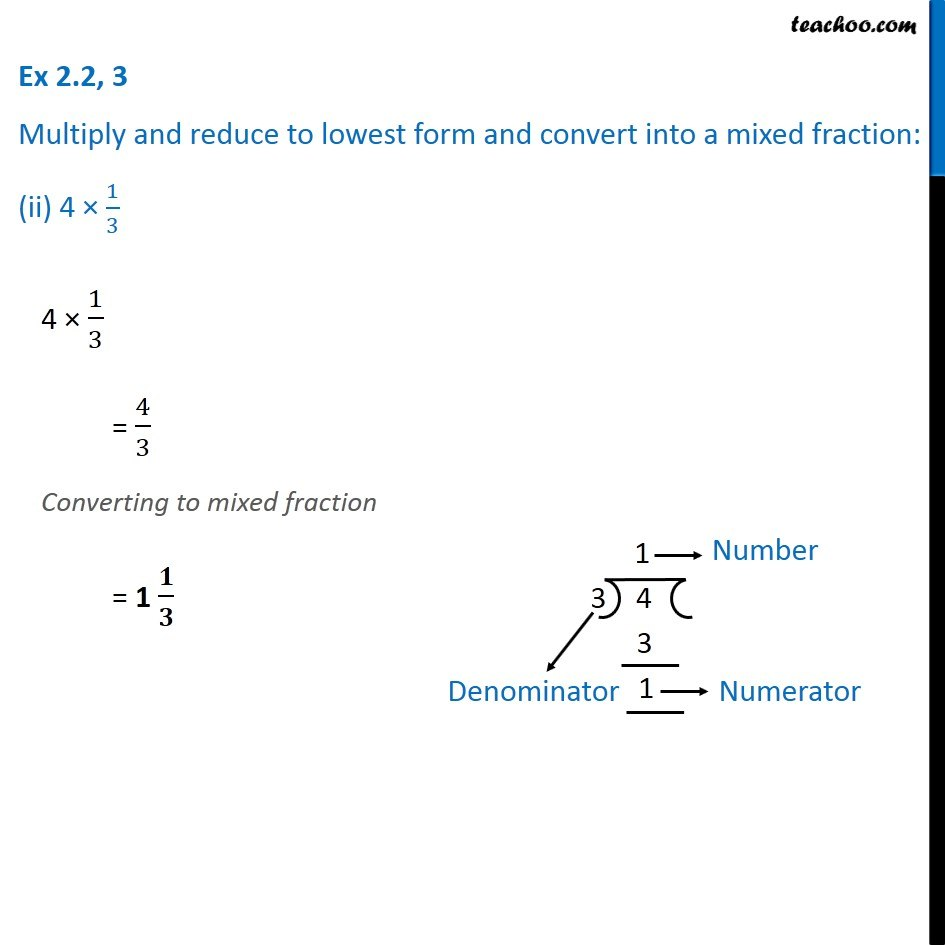Ex 2.2

Chapter 2 Class 7 Fractions and Decimals
Serial order wise### Transcript

Ex 2.2, 3 Multiply and reduce to lowest form and convert into a mixed fraction: (ii) 4 × 1/34 × 1/3 = 4/3 Converting to mixed fraction = 1 𝟏/𝟑 2 × 6/7 = (2 × 6)/7 = 12/7 Converting to mixed fraction = 1 𝟓/𝟕